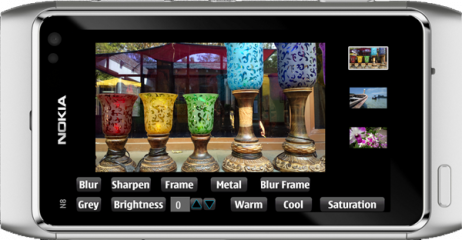2019-07-09 11:32:34 u014570007 阅读数 35
• ###### 实现自己的Prisma（基于QT和OpenCV实现Android平台上...

本课主要内容是基于QT和OpenCV实现Android平台上基于AI的图像处理APP。 重点为QT程序设计、OpenCV.DNN调用深度学习网络和编写完整的Android图像处理程序。 主要基于C++语言，基于QT/QML、OpenCV、torch等基础库和QTcreator。

99 人正在学习 去看看 禾路

### 介绍

.在Qt中有两种表示图像的类，Qt:QImage和QPixmap，还有QBitmap来存储单色的图像，比如遮罩，QPicture在存储QPainter的一些操作指令。

当我们想要在屏幕上绘制图像的时候，最快的方法就是使用QPixmap，不过坏处就是无法访问和修改像素；

QImage在IO操作中有很快的速度，并且给出了访问像素的接口，这篇文章中我们就使用这个类。

.如果你是要处理大的图片，比如摄像头拍摄的照片，这种情况最好是将原图缩小之后作为预览图显示在屏幕上，除非我们允许用户缩放图像。有两种加载并缩放图像的方法。

。将图像加载进QImage或者QPixmap，然后调整大小：

```
```
1. `QImage image("sample.png");`

2. `image = image.scaled(width, height);`

```
```
1. `QImageReader imgReader("sample.png");`

2. `imgReader.setScaledSize(QSize(width, height));`

3. `QImage * image;`

4. `imgReader.read(image);`

。每一张图片都是由像素点组成，每一个像素都有三个通道：红，绿，蓝，还有一个alpha通道来保存透明度（JPEG格式的图片不支持透明）。每个通道的值是0-255，三个通道都是0的话，表示黑色，都是255表示白色。这篇文章中我们用RGB来表示一种颜色，也就是三个通道的值。

。相比于一个像素一个像素地读取，uchar *  QImage::scanLine(int i)可以一次读取整行的像素值，会更加高效，下面的例子就是按行读取的例子，也是我们将要讲的第一个例子，转灰度图。

```
```
1. `QImage * MainWindow::greyScale(QImage * origin){`

2. `QImage * newImage = new QImage(origin->width(), origin->height(), QImage::Format_ARGB32);`

3.
4. `QRgb * line;`

5.
6. `for(int y = 0; y<newImage->height(); y++){`

7. `QRgb * line = (QRgb *)origin->scanLine(y);`

8.
9. `for(int x = 0; x<newImage->width(); x++){`

10. `int average = (qRed(line[x]) + qGreen(line[x]) + qRed(line[x]))/3;`

11. `newImage->setPixel(x,y, qRgb(average, average, average));`

12. `}`

13.
14. `}`

15.
16. `return newImage;`

17. `}`

```
```
1. `QImage * MainWindow::greyScale(QImage * origin){`

2. `QImage * newImage = new QImage(origin->width(), origin->height(), QImage::Format_ARGB32);`

3.
4. `QColor oldColor;`

5.
6. `for(int x = 0; x<newImage->width(); x++){`

7. `for(int y = 0; y<newImage->height(); y++){`

8. `oldColor = QColor(origin->pixel(x,y));`

9. `int average = (oldColor.red()+oldColor.green()+oldColor.blue())/3;`

10. `newImage->setPixel(x,y,qRgb(average,average,average));`

11. `}`

12. `}`

13.
14. `return newImage;`

15. `}`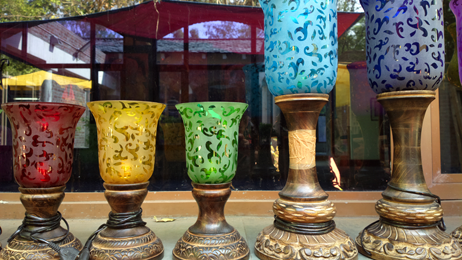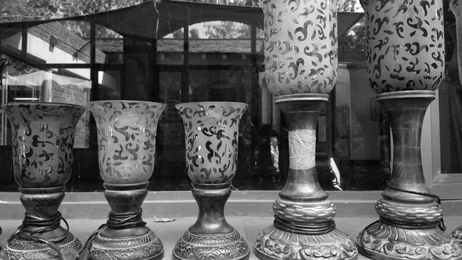### 亮度调节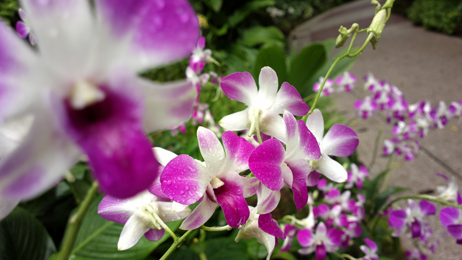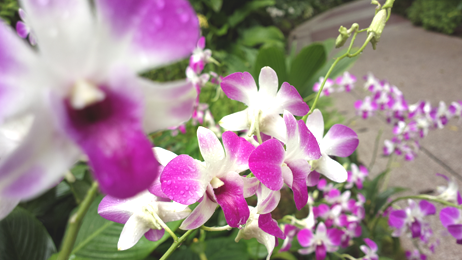### 暖色调

```
```
1. `QImage * MainWindow::warm(int delta, QImage * origin){`

2. `QImage *newImage = new QImage(origin->width(), origin->height(), QImage::Format_ARGB32);`

3.
4. `QColor oldColor;`

5. `int r,g,b;`

6.
7. `for(int x=0; x<newImage->width(); x++){`

8. `for(int y=0; y<newImage->height(); y++){`

9. `oldColor = QColor(origin->pixel(x,y));`

10.
11. `r = oldColor.red() + delta;`

12. `g = oldColor.green() + delta;`

13. `b = oldColor.blue();`

14.
15. `//we check if the new values are between 0 and 255`

16. `r = qBound(0, r, 255);`

17. `g = qBound(0, g, 255);`

18.
19. `newImage->setPixel(x,y, qRgb(r,g,b));`

20. `}`

21. `}`

22.
23. `return newImage;`

24. `}`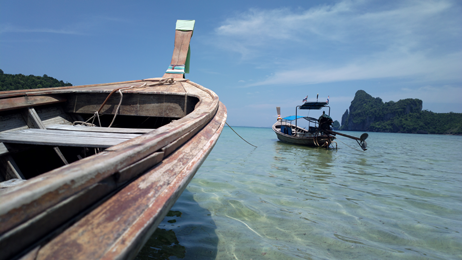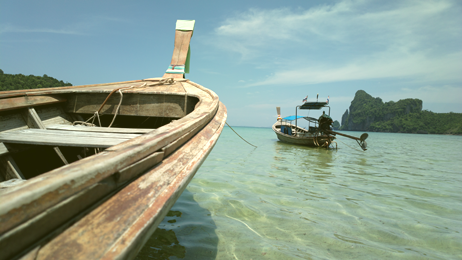### 冷色调

```
```
1. `QImage * MainWindow::cool(int delta, QImage * origin){`

2. `QImage *newImage = new QImage(origin->width(), origin->height(), QImage::Format_ARGB32);`

3.
4. `QColor oldColor;`

5. `int r,g,b;`

6.
7. `for(int x=0; x<newImage->width(); x++){`

8. `for(int y=0; y<newImage->height(); y++){`

9. `oldColor = QColor(origin->pixel(x,y));`

10.
11. `r = oldColor.red();`

12. `g = oldColor.green();`

13. `b = oldColor.blue()+delta;`

14.
15. `//we check if the new value is between 0 and 255`

16. `b = qBound(0, b, 255);`

17.
18. `newImage->setPixel(x,y, qRgb(r,g,b));`

19. `}`

20. `}`

21.
22. `return newImage;`

23. `}`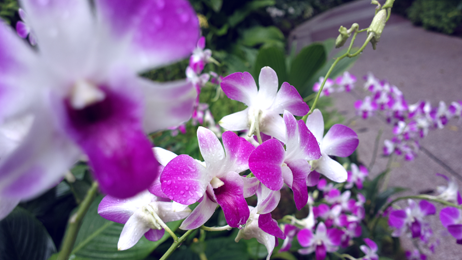### 饱和度

```
```
1. `QImage * MainWindow::saturation(int delta, QImage * origin){`

2. `QImage * newImage = new QImage(origin->width(), origin->height(), QImage::Format_ARGB32);`

3.
4. `QColor oldColor;`

5. `QColor newColor;`

6. `int h,s,l;`

7.
8. `for(int x=0; x<newImage->width(); x++){`

9. `for(int y=0; y<newImage->height(); y++){`

10. `oldColor = QColor(origin->pixel(x,y));`

11.
12. `newColor = oldColor.toHsl();`

13. `h = newColor.hue();`

14. `s = newColor.saturation()+delta;`

15. `l = newColor.lightness();`

16.
17. `//we check if the new value is between 0 and 255`

18. `s = qBound(0, s, 255);`

19.
20. `newColor.setHsl(h, s, l);`

21.
22. `newImage->setPixel(x, y, qRgb(newColor.red(), newColor.green(), newColor.blue()));`

23. `}`

24. `}`

25.
26. `return newImage;`

27. `}`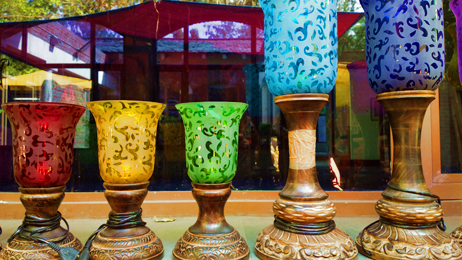### 模糊

R = 20 102 99
150 200 77
170 210 105

G = 22 33 40
17 21 33
8 15 24

B = 88 70 55
90 72 59
85 69 50

Kenel =  0 2 0
2 5 2
0 2 0

r = ( (102*2) + (150*2) + (200*5) + (77*2) + (210*2) ) / (2+2+5+2+2) = 159
g = ( (33*2) + ( 17*2) + (21*5) + (33*2) + (15*2) ) / (2+2+5+2+2) = 23
b = ( (70*2) + (90*2) + (72*5) + (59*2) + (69*2) ) / (2+2+5+2+2) = 72

```
```
1. `QImage * MainWindow::blur(QImage * origin){`

2. `QImage * newImage = new QImage(*origin);`

3.
4. `int kernel = {{0,0,1,0,0},`

5. `{0,1,3,1,0},`

6. `{1,3,7,3,1},`

7. `{0,1,3,1,0},`

8. `{0,0,1,0,0}};`

9. `int kernelSize = 5;`

10. `int sumKernel = 27;`

11. `int r,g,b;`

12. `QColor color;`

13.
14. `for(int x=kernelSize/2; x<newImage->width()-(kernelSize/2); x++){`

15. `for(int y=kernelSize/2; y<newImage->height()-(kernelSize/2); y++){`

16.
17. `r = 0;`

18. `g = 0;`

19. `b = 0;`

20.
21. `for(int i = -kernelSize/2; i<= kernelSize/2; i++){`

22. `for(int j = -kernelSize/2; j<= kernelSize/2; j++){`

23. `color = QColor(origin->pixel(x+i, y+j));`

24. `r += color.red()*kernel[kernelSize/2+i][kernelSize/2+j];`

25. `g += color.green()*kernel[kernelSize/2+i][kernelSize/2+j];`

26. `b += color.blue()*kernel[kernelSize/2+i][kernelSize/2+j];`

27. `}`

28. `}`

29.
30. `r = qBound(0, r/sumKernel, 255);`

31. `g = qBound(0, g/sumKernel, 255);`

32. `b = qBound(0, b/sumKernel, 255);`

33.
34. `newImage->setPixel(x,y, qRgb(r,g,b));`

35.
36. `}`

37. `}`

38. `return newImage;`

39. `}`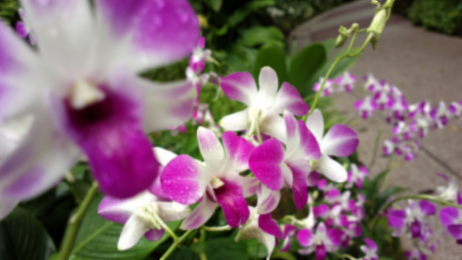### 锐化

```
```
1. `QImage * MainWindow::sharpen(QImage * origin){`

2. `QImage * newImage = new QImage(* origin);`

3.
4. `int kernel = {{0,-1,0},`

5. `{-1,5,-1},`

6. `{0,-1,0}};`

7. `int kernelSize = 3;`

8. `int sumKernel = 1;`

9. `int r,g,b;`

10. `QColor color;`

11.
12. `for(int x=kernelSize/2; x<newImage->width()-(kernelSize/2); x++){`

13. `for(int y=kernelSize/2; y<newImage->height()-(kernelSize/2); y++){`

14.
15. `r = 0;`

16. `g = 0;`

17. `b = 0;`

18.
19. `for(int i = -kernelSize/2; i<= kernelSize/2; i++){`

20. `for(int j = -kernelSize/2; j<= kernelSize/2; j++){`

21. `color = QColor(origin->pixel(x+i, y+j));`

22. `r += color.red()*kernel[kernelSize/2+i][kernelSize/2+j];`

23. `g += color.green()*kernel[kernelSize/2+i][kernelSize/2+j];`

24. `b += color.blue()*kernel[kernelSize/2+i][kernelSize/2+j];`

25. `}`

26. `}`

27.
28. `r = qBound(0, r/sumKernel, 255);`

29. `g = qBound(0, g/sumKernel, 255);`

30. `b = qBound(0, b/sumKernel, 255);`

31.
32. `newImage->setPixel(x,y, qRgb(r,g,b));`

33.
34. `}`

35. `}`

36. `return newImage;`

37. `}`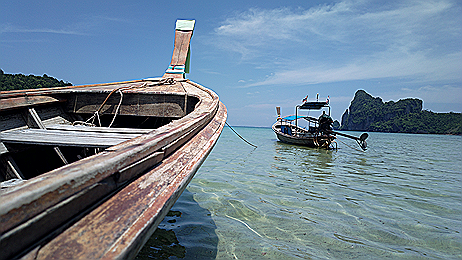### 添加相框

```
```
1. `QImage * MainWindow::drawFrame(QImage * origin){`

2. `QImage * newImage = new QImage(* origin);`

3. `QPainter painter;`

4.
5. `painter.begin(newImage);`

6.
7. `painter.drawImage(0,0, QImage(":images/frame.png"));`

8.
9. `painter.end();`

10.
11. `return newImage;`

12. `}`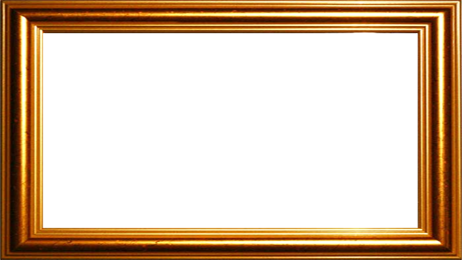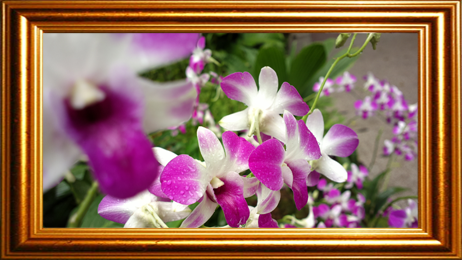### 金属效果

1.调整图像的亮度，获得一个较暗的图片。

2.将图像转成灰度。

3.将灰度图绘制在金属的纹理上，透明度50%。

```
```
1. `QImage * MainWindow::metal(QImage * origin){`

2. `QImage * newImage = new QImage(":images/metal.png");`

3. `QImage * darkImage = brightness(-100, origin);`

4. `QImage * greyImage = greyScale(darkImage);`

5. `QPainter painter;`

6.
7. `painter.begin(newImage);`

8.
9. `painter.setOpacity(0.5);`

10. `painter.drawImage(0, 0, * greyImage);`

11.
12. `painter.end();`

13.
14. `delete greyImage;`

15. `delete darkImage;`

16.
17. `return newImage;`

18. `}`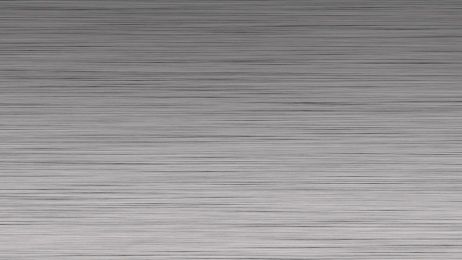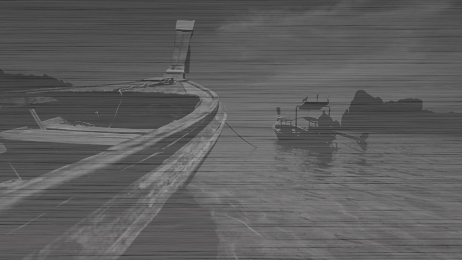### 模糊的边框

1.从原图获取一张完全模糊的图片。

2.使用QPainter的一种融合模式，通过遮罩图片截取出一个模糊的相框。点这里可以学习到更多的QPainter的融合模式。

3.在原图上绘制出模糊的边框。

```
```
1. `QImage * MainWindow::blurFrame(QImage * origin){`

2. `QImage * newImage = new QImage(* origin);`

3. `QImage * blurredImage = blur(newImage);`

4. `QImage * mask = new QImage(":images/mask.png");`

5. `QPainter painter;`

6.
7. `//Using the composition mode SourceAtop we get a blurred frame stored in QImage mask`

8. `painter.begin(mask);`

9.
10. `painter.setCompositionMode(QPainter::CompositionMode_SourceAtop);`

11. `painter.drawImage(0, 0, * blurredImage);`

12.
13. `painter.end();`

14.
15. `//With our new frame we simply draw it over the original image`

16. `painter.begin(newImage);`

17.
18. `painter.setCompositionMode(QPainter::CompositionMode_SourceOver);`

19. `painter.drawImage(0, 0, * mask);`

20.
21. `painter.end();`

22.
23. `delete mask;`

24. `delete blurredImage;`

25.
26. `return newImage;`

27. `}`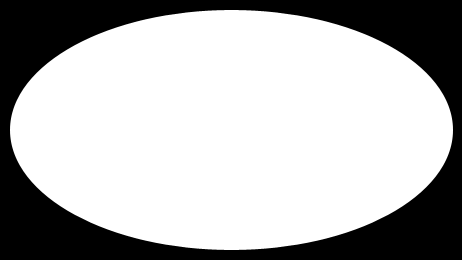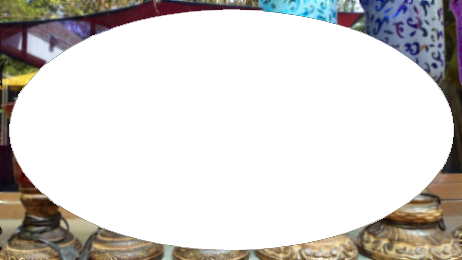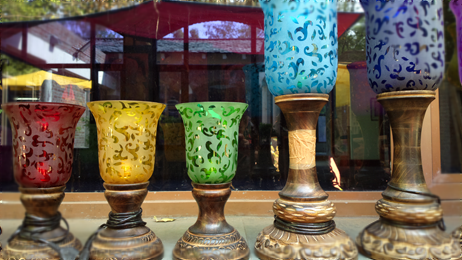### 手机Demo